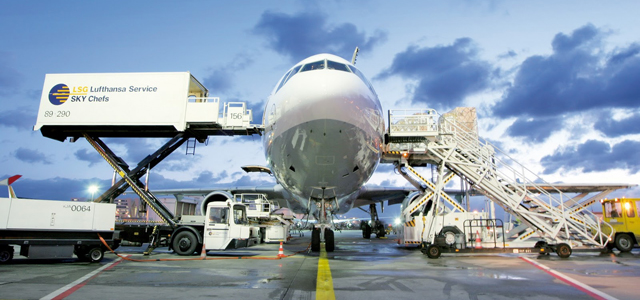# Air Freight Charge Calculation System for Garments Import-Export

### Chargeable Weight Calculation System Under Air Freight for Garments Import-Export

Normally freight charge under air shipment of garments totally depends on both gross weight or actual weight and cargo weight. If the gross weight or actual weight is higher than the cargo weight, then the freight charge is fixed based on gross weight. Again, if the cargo weight is higher than the gross weight, the freight charge is fixed based on cargo weight. That means, among two weights, gross weight and cargo weight, which one will be higher, the freight charge will be fixed on that.Garments Import-Export with Air

Chargeable weight is an equilibrium point, where actual weight and cargo weight are totally balanced. But there is an important reason why freight charge under air shipment totally depends on both gross weight or actual weight and cargo weight.

One example is enough to remove the confusion about it.
Suppose, you export 500kg cotton and another one export 500kg iron under air shipment. Your exported cotton needed more space than iron, but the weight is the same. In this case, if air freight is charged based on cargo weight (based on space) then you have to pay more amount of a charge than an iron exported person. As a result, the actual weight or gross weight and cargo weight both are in consideration during freight charge, which one is higher, that’s chosen for the freight charge.

So, the chargeable weight calculation system in air freight is discussed in the following:
At first, you have to find the cargo measurements with length, width, and height. Then calculate the CBM of cargo by using the following formula-

CBM calculation for cargo = Length (m) ×weight (m) × height (m)

After calculating the CBM of cargo, it’s divided by 0.006. This result is termed as cargo weight. Now, cargo weight is compared to the gross weight or actual weight. Which one is higher, will consider as chargeable weight.

Now I will provide two examples to remove all confusion about the above explanation.

#### Example-01:

Suppose,
Gross weight or actual weight is 200kg,

• Cargo length is 100cm = 1m,
• Cargo width is 100cm = 1m,
• Cargo height is 100cm = 1m.

Find out the chargeable weight for the air freight of this shipment.

Solution:
Now,
We calculate the Cargo CBM = Length (m) ×weight (m) × height (m)
= (1 × 1 × 1) m3
= 1m3

Now, cargo CBM has to divide by 0.006,

Cargo weight = Cargo CBM (m3)/ 0.006
= 1/ 0.006
= 166.67kg.

So, the gross weight or actual weight is 200kg and the cargo weight is 166.67kg. Among the two weights, gross weight or actual weight is higher than cargo weight. As a result, gross weight or actual weight will be chosen for the chargeable weight for this shipment.

#### Example-02:

Suppose,
Gross weight or actual weight is 140kg,

• Cargo length is 98cm = 0.98m,
• Cargo width is 95cm = 0.95m,
• Cargo height is 96cm = 0.96m.

Find out the chargeable weight for the air freight of this shipment.

Solution:
Now,
We calculate the Cargo CBM = Length (m) ×weight (m) × height (m)
= (0.98 × 0.95 × 0.96) m3
= 0.89376 m3

Now, cargo CBM has to divide by 0.006,

Cargo weight = Cargo CBM (m3)/ 0.006
= 0.89376/ 0.006
= 148.96kg.

In this shipment, the gross weight or actual weight is 140kg and the cargo weight is 148.96kg. Among the two weights, cargo weight is higher than gross weight or actual weight. As a result cargo weight will be chosen for the chargeable weight for this shipment.

•lingaraj.b.moji

Very informative
can we know how to calculata the costing of the fabric for a buyer for negitiating the price

•Azgor hossain

Very very thanks…………..sir.
Most important…………valuable.

•GB Linkhon

Dear Maydul vi,
Please give me yarn document tarms 7 detail

•Anwar

Thanks……

This site uses Akismet to reduce spam. Learn how your comment data is processed.